## The FACT Function in Google Sheets (And Why A Shuffled Deck of Cards Is Unique)

Let’s start with a mind-blowing fact, and then use the FACT function in Google Sheets to explain it.

Pick up a standard 52 card deck and give it a good shuffle.

The order of cards in a shuffled deck will be unique.

One that has likely never been seen before in the history of the universe and will likely never be seen again.

I’ll let that sink in.

Isn’t that mind-blowing?

Especially when you picture all the crazy casinos in Las Vegas.

Let’s understand why, and in the process learn about the FACT function and basic combinatorics (the study of counting in mathematics).

## Four Card Deck

To keep things simple, suppose you only have 4 cards in your deck, the four aces.

You can create this deck in Google Sheets with the CHAR function: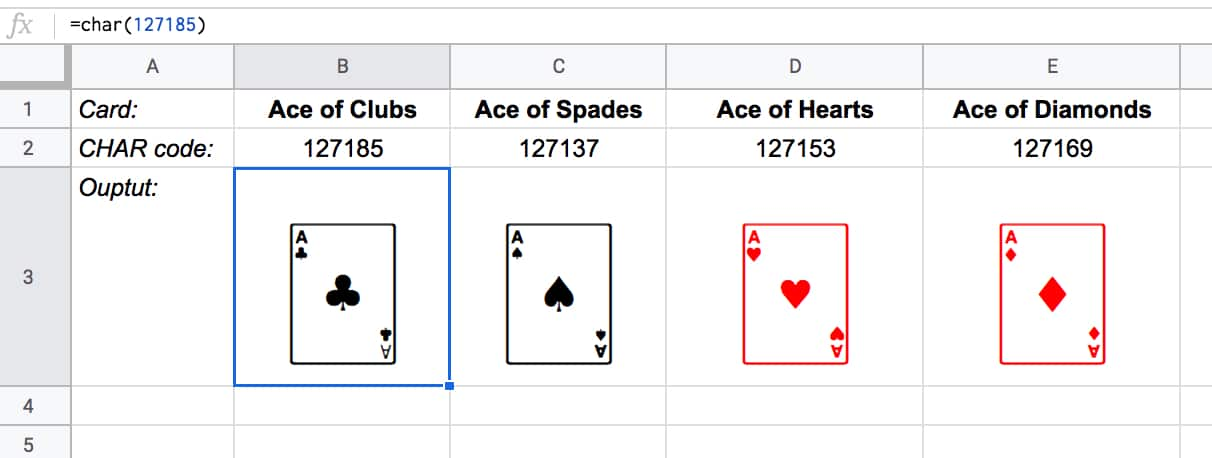The formulas to create these four cards are:

Ace of Clubs:

`=CHAR(127185)`

`=CHAR(127137)`

Ace of Hearts:

`=CHAR(127153)`

Ace of Diamonds:

`=CHAR(127169)`

Let’s see how many different combinations exist with just these four cards.

Pick one of them to start. You have a choice of four cards at this stage.

Once you’ve chosen the first one, you have three cards left, so there are 3 possible options for the second card choice.

When you’ve picked that second card, you have two cards left. So you have a choice of two for the third card.

The final card is the last remaining one.

So you have 4 choices * 3 choices * 2 choices * 1 choice = 4 * 3 * 2 * 1 = 24

There are 24 permutations (variations) with just 4 cards!

Visually, we can show this in our Google Sheet by displaying all the different combinations with the card images from above: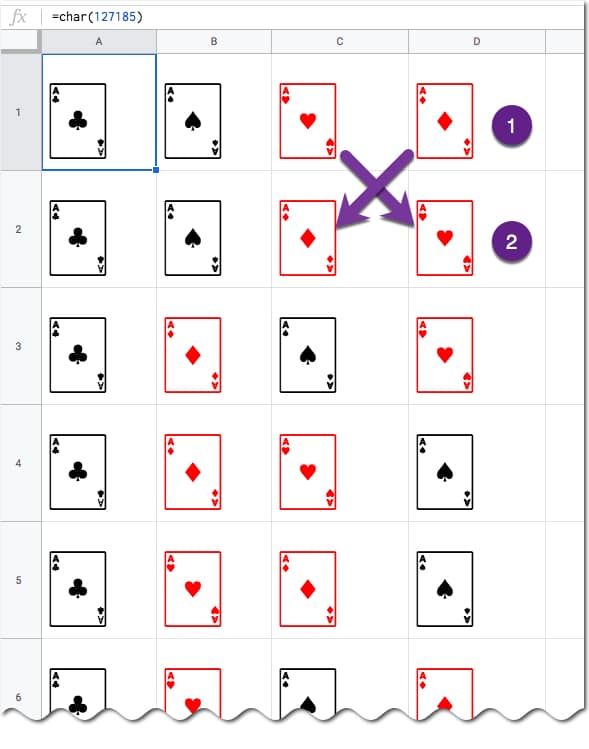(I’ve just shown the first 6 rows for brevity.)

You can see for example, when moving from row 1 to row 2, we swapped the position of the two red suits: the Ace of Hearts and the Ace of Diamonds.

## Five Card Deck

This time there are 5 choices for the first card, then 4, then 3, then 2, and finally 1.

So the number of permutations is 5 * 4 * 3 * 2 * 1 = 120

Already a lot more! I have not drawn this out in a Google Sheet and leave that as an optional exercise for you if you wish.

## The FACT function

The FACT function in Google Sheets (see documentation) is a math function that returns the factorial of a given number. The factorial is the product of that number with all the numbers lower than it down to 1.

In other words, exactly what we’ve done above in the above calculations.

Four:

The 4 card deck formula is:

=FACT(4)

which gives an answer of 24 permutations.

Five:

The 5 card deck formula is:

=FACT(5)

which gives an answer of 120 permutations.

Six:

A 6 card deck is:

=FACT(6)

which gives an answer of 720 permutations.

Twelve:

A 12 card deck has  479,001,600 different ways of being shuffled:

=FACT(12)

(You’re more likely to win the Powerball lottery at 1 in 292 million odds than to get two matching shuffled decks of cards, even with just 12 cards!)

Fifty Two:

Keep going up to a full deck of 52 cards with the formula and it’s a staggeringly large number.

=FACT(52)

Type it into Google Sheets and you’ll see an answer of 8.07E+67, which is 8 followed by 67 zeros!

(This number notation is called scientific notation, where huge numbers are rounded to the first few digits multiplied by a 10 to the power of some number, 67 in this case.)

This answer is more than the number of stars in the universe (about 10 followed by 21 zeros).

Put another way if all 6 billion humans on earth began shuffling cards at 1 deck per minute every day of the year for millions of years, we still wouldn’t even be close to finding all possible combinations.

## Build Numbered Lists With The Amazing SEQUENCE Function

The SEQUENCE function is a useful function in Google Sheets. It’s a powerful way to generate numbered lists.

Previously, you had to resort to obscure array formulas like

`=ArrayFormula(row(1:5))`

to get lists of numbers. Things got ugly fast if you wanted to customize these lists.

Thankfully today, we have the SEQUENCE function.

As arguments, you specify: 1) the number of rows, 2) the number of columns, 3) a start value, and 4) a step size.

Arguments 2, 3 and 4 are optional. However, if you want to set them you need to include the previous ones (e.g. if you want to set a step size in argument 4, then you need to set 1, 2 and 3 as well).

Keep this order in mind as you look through the examples below and you’ll soon understand how the function works.

## 1. Ascending list of numbers

`=SEQUENCE(5)`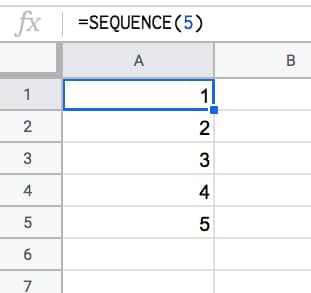## 2. Horizontal list of numbers

Set the row count to 1 and the column count to however many numbers you want e.g. 5:

`=SEQUENCE(1,5)`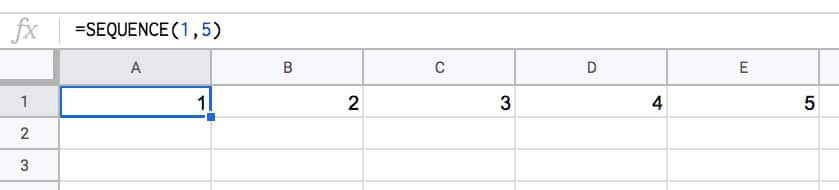## 3. Two-dimensional array of numbers

Set both row and number values:

`=SEQUENCE(10,5)`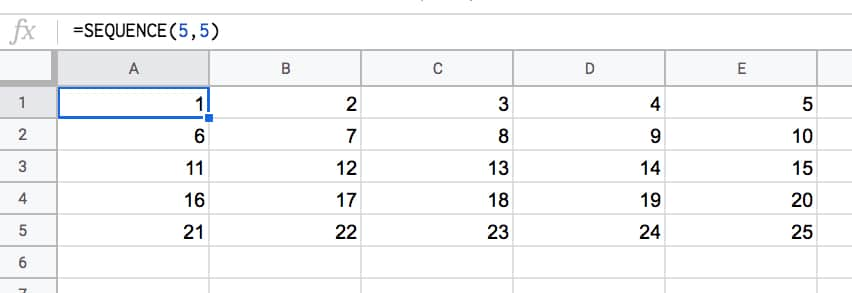## 4. Start from a specific value

Set the third argument to the value you want to start from e.g. 100:

`=SEQUENCE(5,1,100)`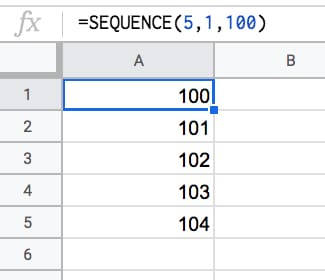## 5. Use a custom step

Set the fourth argument to the size of the step you want to use, e.g. 10:

`=SEQUENCE(5,1,1,10)`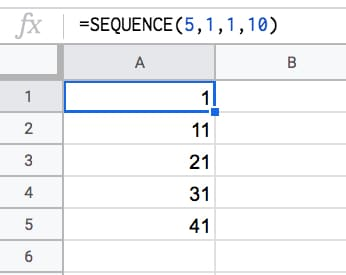## 6. Descending numbers

Set the fourth argument to -1 to count down:

`=SEQUENCE(5,1,5,-1)`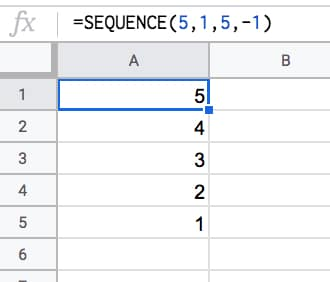## 7. Negative numbers

Set the start value to a negative number and/or count down with negative step:

`=SEQUENCE(5,1,-1,-1)`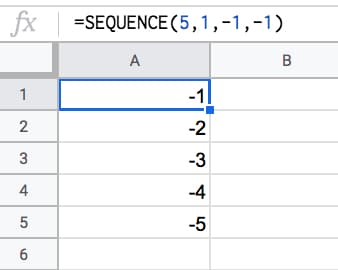## 8. Dates

Dates are stored as numbers in spreadsheets, so you can use them inside the SEQUENCE function. You need to format the column as dates:

`=SEQUENCE(5,1,TODAY(),1)`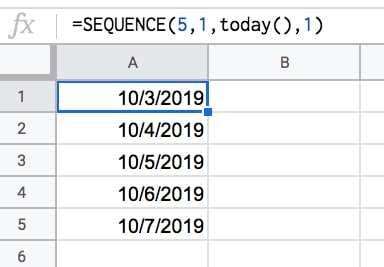## 9. Decimal numbers

Unfortunately you can’t set decimal counts directly inside the SEQUENCE function, so you have to combine with an Array Formula e.g.

`=ArrayFormula( SEQUENCE(5,1,10,1) / 10 )`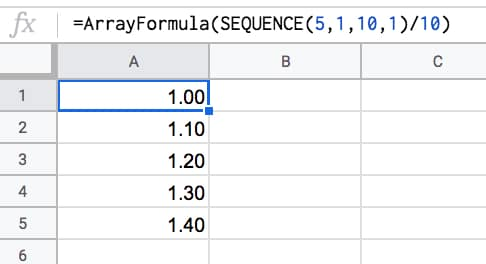## 10. Constant numbers

You’re free to set the step value to 0 if you want an array of constant numbers: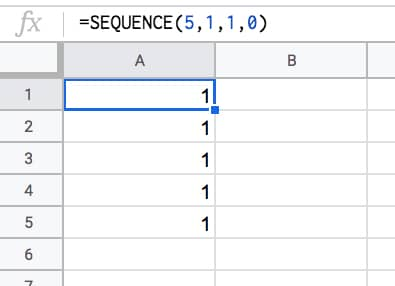## 11. Monthly sequences

Start with this formula in cell A1, which gives the numbers 1 to 12 in a column:

`=SEQUENCE(12)`

In the adjacent column, use this DATE function to create the first day of each month (formula needs to be copied down all 12 rows):

`=DATE(2021,A1,1)`

This can be turned into an Array Formula in the adjacent column, so that a single formula, in cell C1, outputs all 12 dates:

`=ArrayFormula(DATE(2021,A1:A12,1))`

Finally, the original SEQUENCE formula can be nested in place of the range reference, using this formula in cell D1:

`=ArrayFormula(DATE(2021,SEQUENCE(12),1))`

This single formula gives the output:

1/1/2021
2/1/2021
.
.
.
12/1/2021

It’s an elegant way to create a monthly list. It’s not dependent on any other input cells either (columns A, B, C are working columns in this example).

With this formula, you can easily change all the dates, e.g. to 2022.

Building in steps like this a great example of the Onion Method, which I advocate for complex formulas.

## 12. Text and Emoji sequences

You can use a clever trick to set the SEQUENCE output to a blank string using the TEXT function. Then you can append on a text value or an emoji or whatever string you want to create a text list.

For example, this repeats the name “Ben Collins” one hundred times in a column:

`=ArrayFormula(TEXT(SEQUENCE(100,1,1,1),"")&"Ben Collins")`

And, by using the CHAR function, you can also make emoji lists. For example, here’s a 10 by 10 grid of tacos:

`=ArrayFormula(TEXT(SEQUENCE(10,10,1,1),"")&CHAR(127790))`Have you got any examples of using the SEQUENCE function?

## Unpivot In Google Sheets With Formulas (How To Turn Wide Data Into Tall Data)

Unpivot in Google Sheets is a method to turn “wide” tables into “tall” tables, which are more convenient for analysis.

Suppose we have a wide table like this: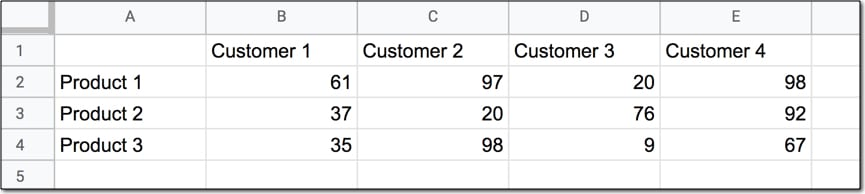Wide data like this is good for the Google Sheets chart tool but it’s not ideal for creating pivot tables or doing analysis. The main reason is that data is captured in the column headings, which prevents you using it in pivot tables for analyis.

So we want to transform this data — unpivot it — into the tall format that is the way databases store data: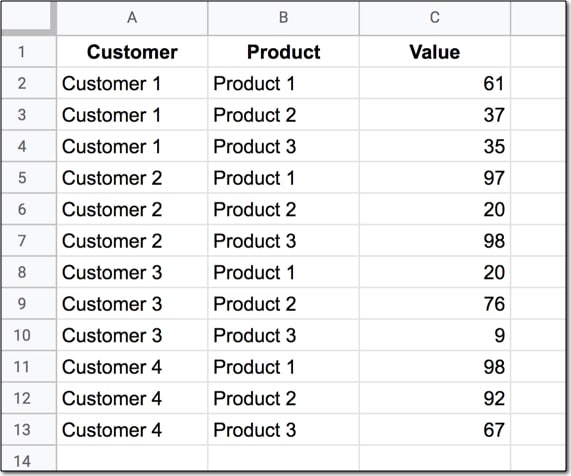But how do we unpivot our data like that?

It turns out it’s quite hard.

It’s harder than going the other direction, turning tall data into wide data tables, which we can do with a pivot table.

This article looks at how to do it using formulas so if you’re ready for some complex formulas, let’s dive in…

We’ll use the wide dataset shown in the first image at the top of this post.

The output of our formulas should look like the second image in this post.

In other words, we need to create 16 rows to account for the different pairings of Customer and Product, e.g. Customer 1 + Product 1, Customer 1 + Product 2, etc. all the way up to Customer 4 + Product 4.

Of course, we’ll employ the Onion Method to understand these formulas.

### Template

Feel free to make your own copy (File > Make a copy…).

(If you can’t open the file, it’s likely because your G Suite account prohibits opening files from external sources. Talk to your G Suite administrator or try opening the file in an incognito browser.)

### Step 1: Combine The Data

Use an array formula like this to combine the column headings (Customer 1, Customer 2, etc.) with the row headings (Product 1, Product 2, Product 3, etc.) and the data.

It’s crucial to add a special character between these sections of the dataset though, so we can split them up later on. I’ve used the fox emoji (because, why not?) but you can use whatever you like, provided it’s unique and doesn’t occur anywhere in the dataset.

`=ArrayFormula(B1:E1&"🦊"&A2:A4&"🦊"&B2:E4)`

The output of this formula is: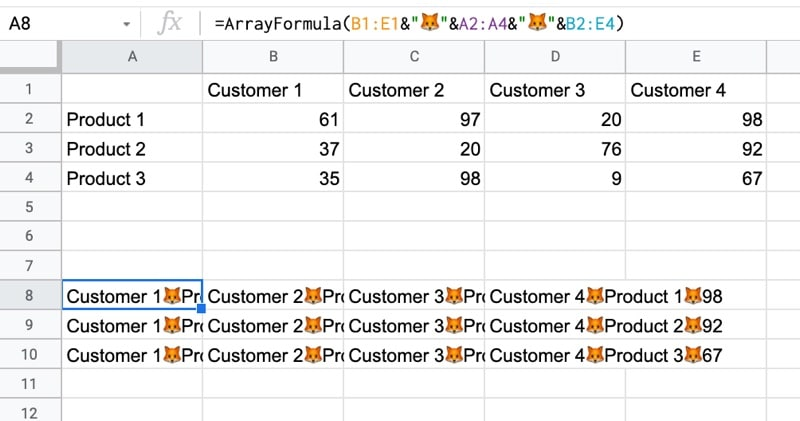### Step 2: Flatten The Data

Before the introduction of the FLATTEN function, this step was much, much harder, involving lots of weird formulas.

Thankfully the FLATTEN function does away with all of that and simply stacks all of the columns in the range on top of each other. So in this example, our combined data turns into a single column.

`=ArrayFormula(FLATTEN(B1:E1&"🦊"&A2:A4&"🦊"&B2:E4))`

The result is: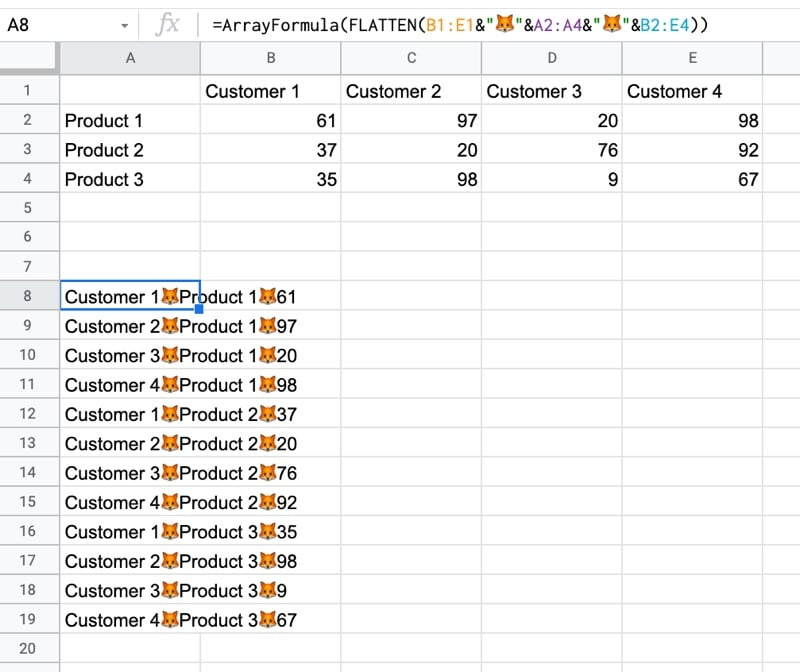### Step 3: Split The Data Into Columns

The final step is to split this new tall column into separate columns for each data type. You can see now why we needed to include the fox emoji so that we have a unique character to split the data on.

Wrap the formula from step 2 with the SPLIT function and set the delimiter to “🦊”:

`=ArrayFormula(SPLIT(FLATTEN(B1:E1&"🦊"&A2:A4&"🦊"&B2:E4),"🦊"))`

This splits the data into the tall data format we want. All that’s left is to add the correct column headings.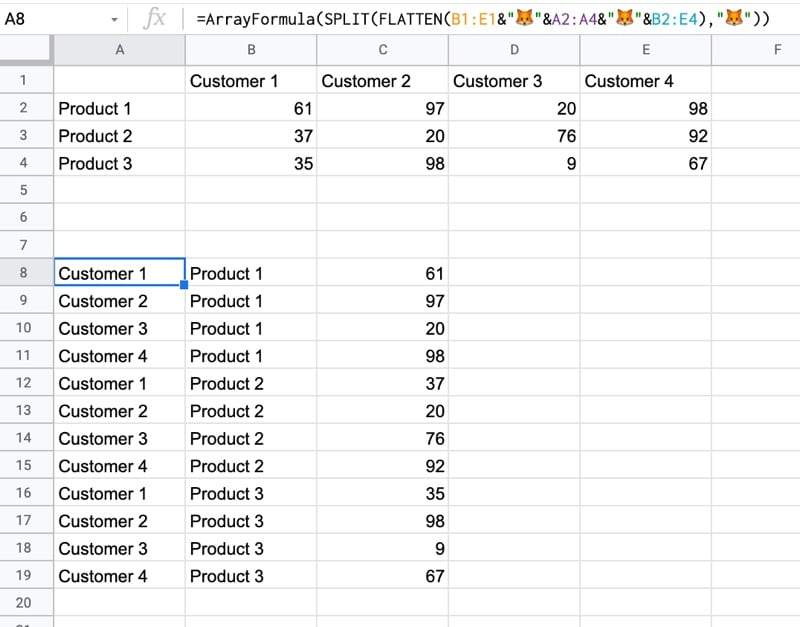## Unpivot With Apps Script

You can also use Apps Script to unpivot data.

Have a look at the example sheet from the first answer of this Stack Overflow post.

## Formula Challenge #5: Merge Columns in Google Sheets With Single Range Reference

This Formula Challenge originally appeared as Tip #131 of my weekly Google Sheets Tips newsletter, on 21 December 2020. Sign up so you don’t miss future Formula Challenges!

Find all the Formula Challenges archived here.

## The Challenge: Merge Columns With Single Range Reference

Question: How can you merge n number of columns and get the unique values, without typing each one out?

In other words, can you create a single formula that gives the same output as this one:

`=SORT( UNIQUE( {A:A;B:B;C:C;...;XX:XX} )) `

but without having to write out A:A, B:B, C:C, D:D etc. and instead just write A:XX as in the input?

Use this simple dataset example, where your formula will be in cell E1 (in green):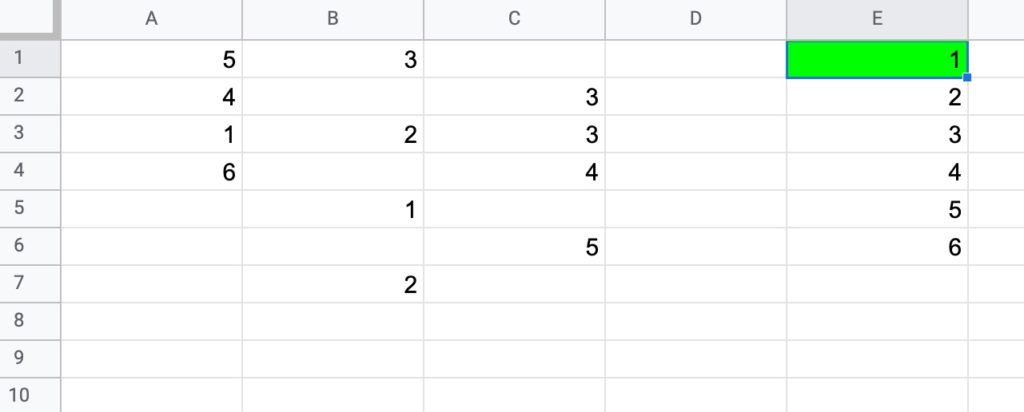• be a formula in a single cell
• work with the input range in the form A:XX (e.g. A:C in this example)
• work with numbers and/or text values
• return only the unique values, in an ascending order in a SINGLE COLUMN.

## Solutions To Sort A Column By Last Name

I received 67 replies to this formula challenge with two different methods for solving it. Congratulations to everyone who took part!

I learnt so much from the different replies, many of which proffered a shorter and more elegant second solution than my own original formula.

Here I present the two solutions.

There’s a lot to learn by looking through them.

### 1. FLATTEN method

`=SORT(UNIQUE(FLATTEN(A:C)))`

Flatten collects all the data from the ranges into a single column, before the unique ones are selected and then sorted.

The FLATTEN function is a new function that popped up in early 2020, but was only recently documented by Google.

It’s a powerful formula, and one that can be used to unpivot data.

Note 1: you can have multiple inputs (arguments) to the FLATTEN function. Data is ordered by the order of the inputs, then row and then column.

Note 2: at the moment the FLATTEN function doesn’t show up in the auto-complete when you start typing it out. You can still use it, but you’ll have to type it out fully yourself.

Thanks to the handful of you that shared this neat solution with me. Great work!

### 2. TEXTJOIN method

Join all the values in A:C with TEXTJOIN, using a unique character as the delimiter (in this case, the King and Queen chess pieces!)

You want to use an identifier that is not in columns A to C.

`=TEXTJOIN("♔♕",TRUE,A:C)`

Split on this unique delimiter using the SPLIT function:

`=SPLIT(TEXTJOIN("♔♕",TRUE,A:C),"♔♕")`

Transpose to a column, select the unique values only and finally wrap with a sort function to get the result:

`=SORT(UNIQUE(TRANSPOSE(SPLIT(TEXTJOIN("♔♕",TRUE,A:C),"♔♕"))))`

There we go!

Two brilliant solutions to an interesting formula challenge.